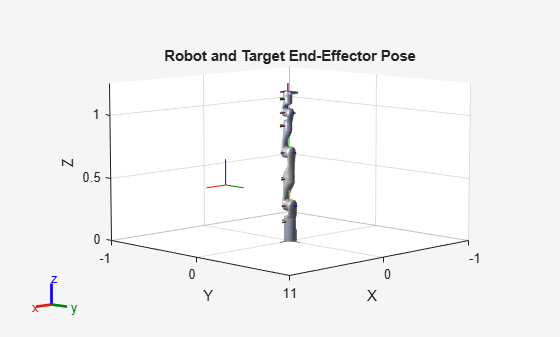Model rigid body tree motion given task-space reference inputs

Since R2019b

## Description

The `taskSpaceMotionModel` object models the closed-loop task-space motion of a manipulator, specified as a rigid body tree object. The motion model behavior is defined by the MotionType property.

For more details about the equations of motion, see Task-Space Motion Model.

## Creation

### Syntax

``motionModel = taskSpaceMotionModel``
``motionModel = taskSpaceMotionModel("RigidBodyTree",tree)``
``motionModel = taskSpaceMotionControlModel(Name,Value)``

### Description

````motionModel = taskSpaceMotionModel` creates a motion model for a default two-joint manipulator.```
````motionModel = taskSpaceMotionModel("RigidBodyTree",tree)` creates a motion model for the specified `rigidBodyTree` object.```

example

````motionModel = taskSpaceMotionControlModel(Name,Value)` sets additional properties specified as name-value pairs. You can specify multiple properties in any order.```

## Properties

expand all

Rigid body tree robot model, specified as a `rigidBodyTree` object that defines the inertial and kinematic properties of the manipulator.

This property defines the body that will be used as the end effector, and for which the task-space motion is defined. The property must correspond to a body name in the `rigidBodyTree` object of the RigidBodyTree property. If the rigid body tree is updated without also updating the end effector, the body with the highest index becomes the end-effector body by default.

Proportional gain for PD control, specified as a 6-by-6 matrix.

Derivative gain for proportional-derivative (PD) control, specified as a 6-by-6 matrix.

Joint damping constant, specified as an n-element vector, where n is the number of non-fixed joints in the robot model specified by the RigidBodyTree property. Joint damping units are N/(m/s) or N/(rad/s) for prismatic and revolute joints, respectively.

Type of motion, specified as `"PDControl"`, which uses proportional-derivative (PD) control mapped to the joints via a Jacobian-Transpose controller. The control is based on the specified Kp and Kd properties.

To use computed torque control or independent joint motion, use the `jointSpaceMotionModel` object.

## Object Functions

 `derivative` Time derivative of manipulator model states `updateErrorDynamicsFromStep` Update values of `NaturalFrequency` and `DampingRatio` properties given desired step response

## Examples

collapse all

This example shows how to create and use a `taskSpaceMotionModel` object for a manipulator robot arm in task-space.

Create the Robot

`robot = loadrobot("kinovaGen3","DataFormat","column","Gravity",[0 0 -9.81]);`

Set Up the Simulation

Set the time span to be 1 second with a timestep size of 0.02 seconds. Set the initial state to the home configuration of the robot, with a velocity of zero.

```tspan = 0:0.02:1; initialState = [homeConfiguration(robot);zeros(7,1)];```

Define a reference state with a target position and zero velocity.

```refPose = trvec2tform([0.6 -.1 0.5]); refVel = zeros(6,1);```

Create the Motion Model

Model the behavior as a system under proportional-derivative (PD) control.

`motionModel = taskSpaceMotionModel("RigidBodyTree",robot,"EndEffectorName","EndEffector_Link");`

Simulate the Robot

Simulate the behavior over 1 second using a stiff solver to more efficiently capture the robot dynamics`.` Using `ode15s` enables higher precision around the areas with a high rate of change.

`[t,robotState] = ode15s(@(t,state)derivative(motionModel,state,refPose,refVel),tspan,initialState);`

Plot the Response

Plot the robot's initial position and mark the target with an X.

```figure show(robot,initialState(1:7)); hold all plot3(refPose(1,4),refPose(2,4),refPose(3,4),"x","MarkerSize",20)```

Observe the response by plotting the robot in a 5 Hz loop.

```r = rateControl(5); for i = 1:size(robotState,1) show(robot,robotState(i,1:7)',"PreservePlot",false); waitfor(r); end```Craig, John J. Introduction to Robotics: Mechanics and Control. Upper Saddle River, NJ: Pearson Education, 2005.

 Spong, Mark W., Seth Hutchinson, and Mathukumalli Vidyasagar. Robot Modeling and Control. Hoboken, NJ: Wiley, 2006.